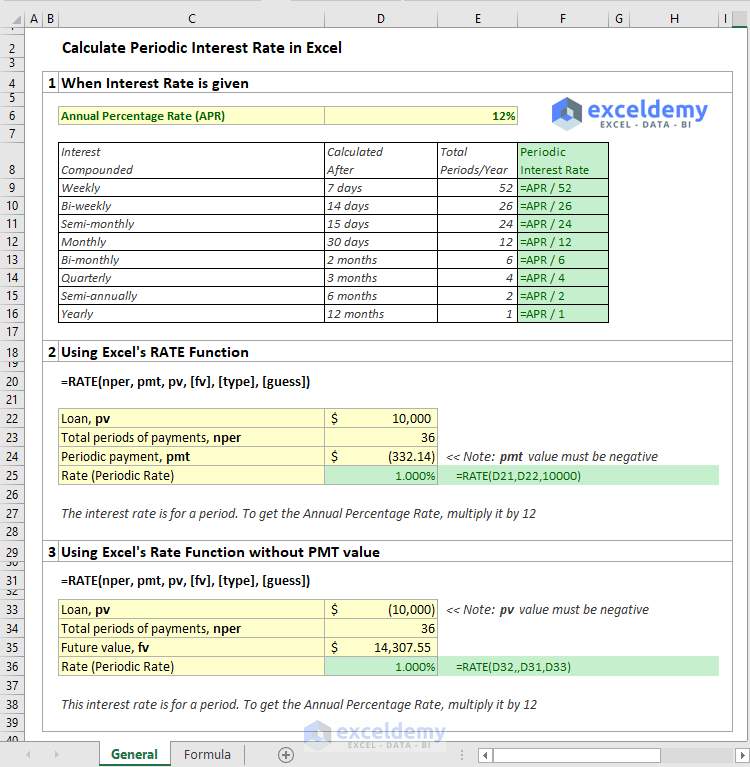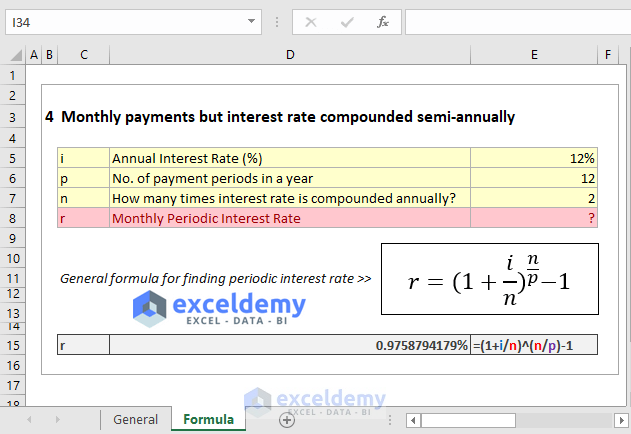Disclosure: This post may contain affiliate links, meaning when you click the links and make a purchase, we receive a commission.

# How to calculate periodic interest rate in Excel (4 ways)In this short article, I will show how to calculate periodic interest rate in Excel. I will show 4 methods of calculating the periodic interest rate.

## 1) Calculate periodic interest rate when the interest rate is given

You can pay the repayments of a loan weekly, bi-weekly, semi-monthly, monthly, bi-monthly, quarterly, semi-annually, or yearly.

From the following table, you can find the periodic interest rate from yearly interest rate by dividing the yearly interest rate by total No. of Periods / Year.

 Interest Compounded Calculated After No. of Periods / Year Periodic Interest Rate Weekly 7 days 52 =APR/52 Bi-weekly 14 days 26 =APR/26 Semi-monthly 15 days 24 =APR/24 Monthly 30 days 12 =APR/12 Bi-monthly 2 months 6 =APR/6 Quarterly 3 months 4 =APR/4 Semi-annually 6 months 2 =APR/2 Yearly 12 months 1 =APR/1

## 2) Periodic Interest Rate using Excel’s RATE Function

Syntax of Excel’s RATE Function: =RATE(nper, pmt, pv, [fv], [type], [guess])

Say, you took a loan of \$10,000 for 3 years. Your monthly payment is \$332.14. What is your monthly periodic interest rate or yearly interest rate?

Loan, pv = \$10,000

Total no. of periods for payments, nper = 3 years x 12 = 36

Periodic payment, pmt = -\$332.14

Rate (Periodic Rate) = RATE(36, -332.14, 10000) = 1%

So, the monthly interest rate is 1%.

Then, the yearly interest rate is 1% x 12 = 12%

## 3) Periodic Interest Rate using Excel’s RATE Function without PMT value

This time, the monthly payment is not given. But the future value of your loans is given.

Loan, pv = \$10,000

Total periods of payments, nper = 36

Future value, fv = \$14,307.55

Rate (Periodic Rate) = RATE(36, , 10000, 14307.55) = 1%

As the pmt value is not given, we have kept a blank space for this argument in the RATE formula.

## 4) Your payment is monthly but interest is compounded Semi-annually. What will be your periodic interest rate?

You have to use this formula: r = (1 + i/n)^(n/p) – 1

Here,

r = Interest rate for per payment period

i = Annual Interest Rate (%)

n = number of compounding periods per year

p = number of payments per year

If APR (annual interest rate) is 12%, interest rate (i) is compounded semi-annually (n = 2), but you have to pay monthly, then your periodic interest rate,

r = (1 + 12%/2)^(2/12)-1 = (1+6%)^(1/6) – 1 = 0.97588%

This is a general formula for finding the periodic interest rate.